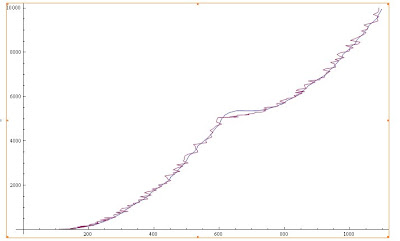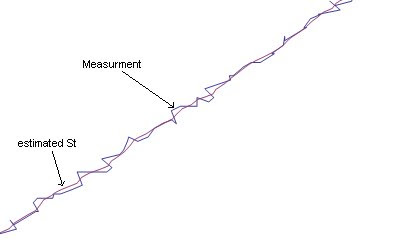## Monday, April 20, 2009

### State estimation using Kalman filter

Kalman filter helps to find the correct state of a linear system , provided the approximate state of the system at time 't'. It has wide application in the following fields  Aerospace,computer vision, signal processing etc.

Let 'St' be the state variable at time t

St1 = A * St0 + V  ,
V is the random noise with mean = 0
A is the gain Matrix of the system.

Let Mt be the measurment variable. Measurment is the approxiamte state at time t. Kalman filter can predict the correct state from this measurment( also depending on other params etc  ).

Mt1 = H * St1 + W
H is the gain Matrix.
W is the random noise with mean = 0.

The interseting thing is usually Mt1 is known at time t1 and we want to find the st1 which is the correcte state at time t1 from this Mt1.

Kalman filter uses kalman gain to compute the state St.
St = St + Kt*( Mt - H*St ) , Kt is the gain matrix. and the new St is calculatd using the kalman gain Kt.

The noise is represented in this system using a covariace matrix. Let P represent the noise of function V ( system noise )  and R represent the noise during measurment.

I am omitting the detailed derivation of equations here. The final equation and steps are shown below.
At time 't' do the follwing to estimate the correct state St.

Update the state vector and measurment covariance matrix.
St = A * St
P =  A * P * Transpose[P]

find the kalman gain for this.
K = A*P0*Transpose[H]* Inverse[  ( H*P0*Transpose[H] + R) ]

Mt = Do measurment here and assign to Mt.

Calcualte the estimated state St.
St = St + K* ( Mt - H*St );
Finally update the state updation covariance matrix P
P = P - K*(H*P )

Repeat these steps for each measurment.Following figure shows the result.
The  measurment data 'Mt' , which is affected by noise.and rectified data St which is the output of Kalman filter and it gives us better prediction of the state.